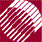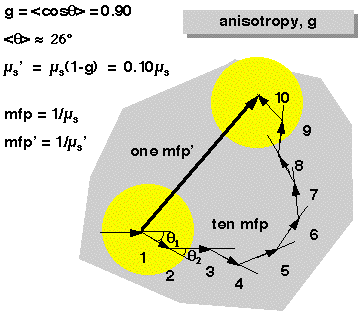ECE532 Biomedical Optics © 1998 Steven L. Jacques, Scott A. Prahl Oregon Graduate Institute

# Reduced scattering coefficient

The reduced scattering coefficient is a lumped property incorporating the scattering coefficient µs and the anisotropy g:

µs' = µs(1 - g) [cm-1]

The purpose of µs' is to describe the diffusion of photons in a random walk of step size of 1/µs' [cm] where each step involves isotropic scattering. Such a description is equivalent to description of photon movement using many small steps 1/µs that each involve only a partial deflection angle θ if there are many scattering events before an absorption event, i.e., µa << µs'. This situation of scattering-dominated light transport is called the diffusion regime and µs'is useful in the diffusion regime which is commonly encountered when treating how visible and near-infrared light propagates through biological tissues.

The following figure shows the equivalence of taking 10 smaller steps of "mean free path" mfp = 1/µs with anisotropic deflection angles and one big step with a "reduced mean free path" mfp' = 1/µs'.The following figure shows how many such big steps involving isotropic scattering are equivalent to many small anisotropic steps: